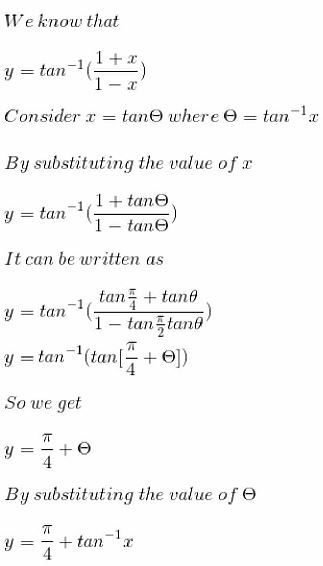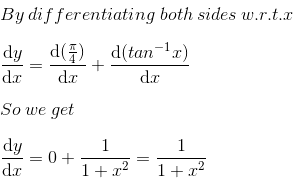# RS Aggarwal Solutions for Class 12 Chapter 10: Differentiation Exercise 10D

This exercise covers major topics like differentiation by trigonometric transformations along with solved examples. Solving the exercise wise problems might be difficult for students during the exam preparation. To boost their confidence in solving problems, the subject experts at BYJU’S have prepared solutions in a simple way. To obtain a better academic score, students can use RS Aggarwal Solutions Class 12 Maths Chapter 10 Differentiation Exercise 10D PDF from the links given here.

## RS Aggarwal Solutions for Class 12 Maths Chapter 10: Differentiation Exercise 10D Download PDF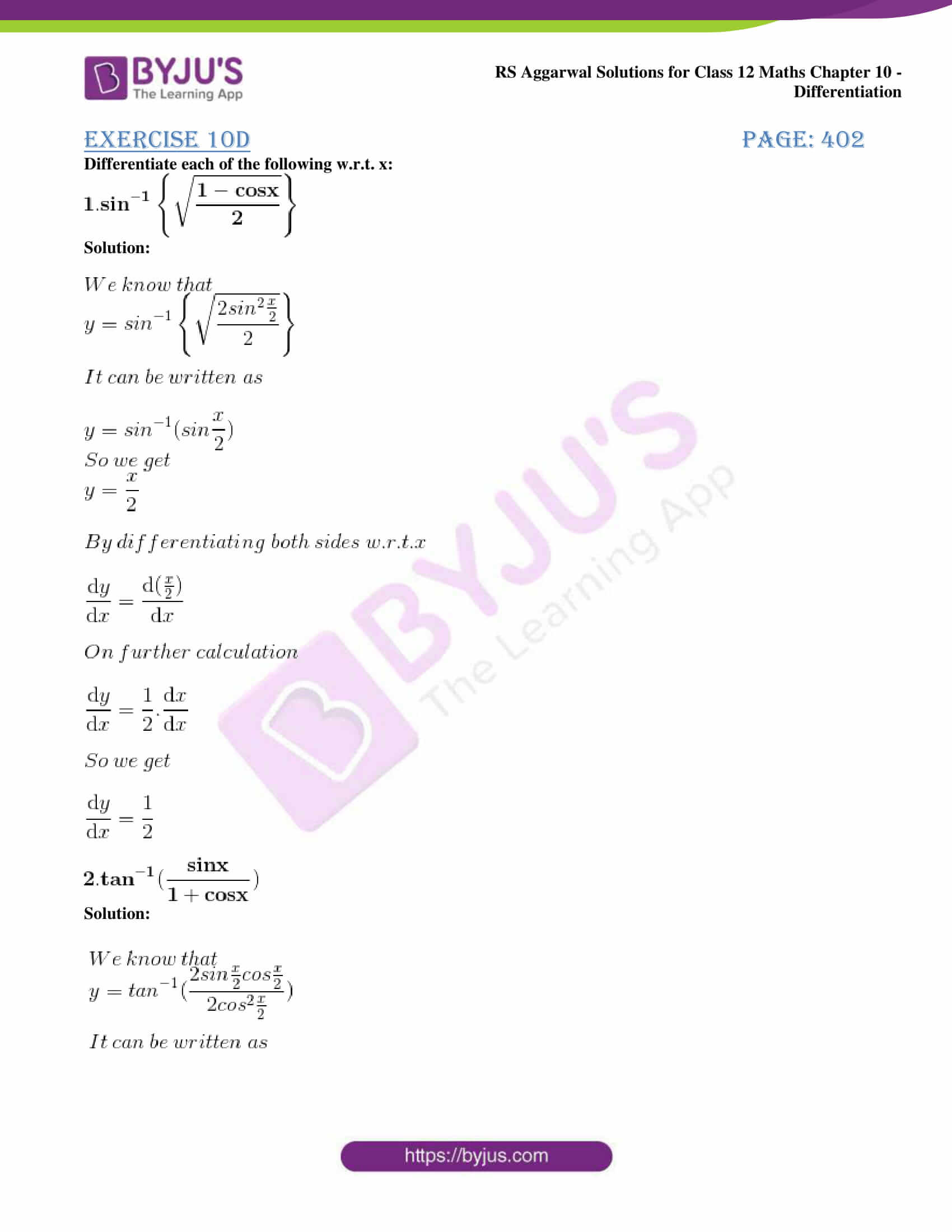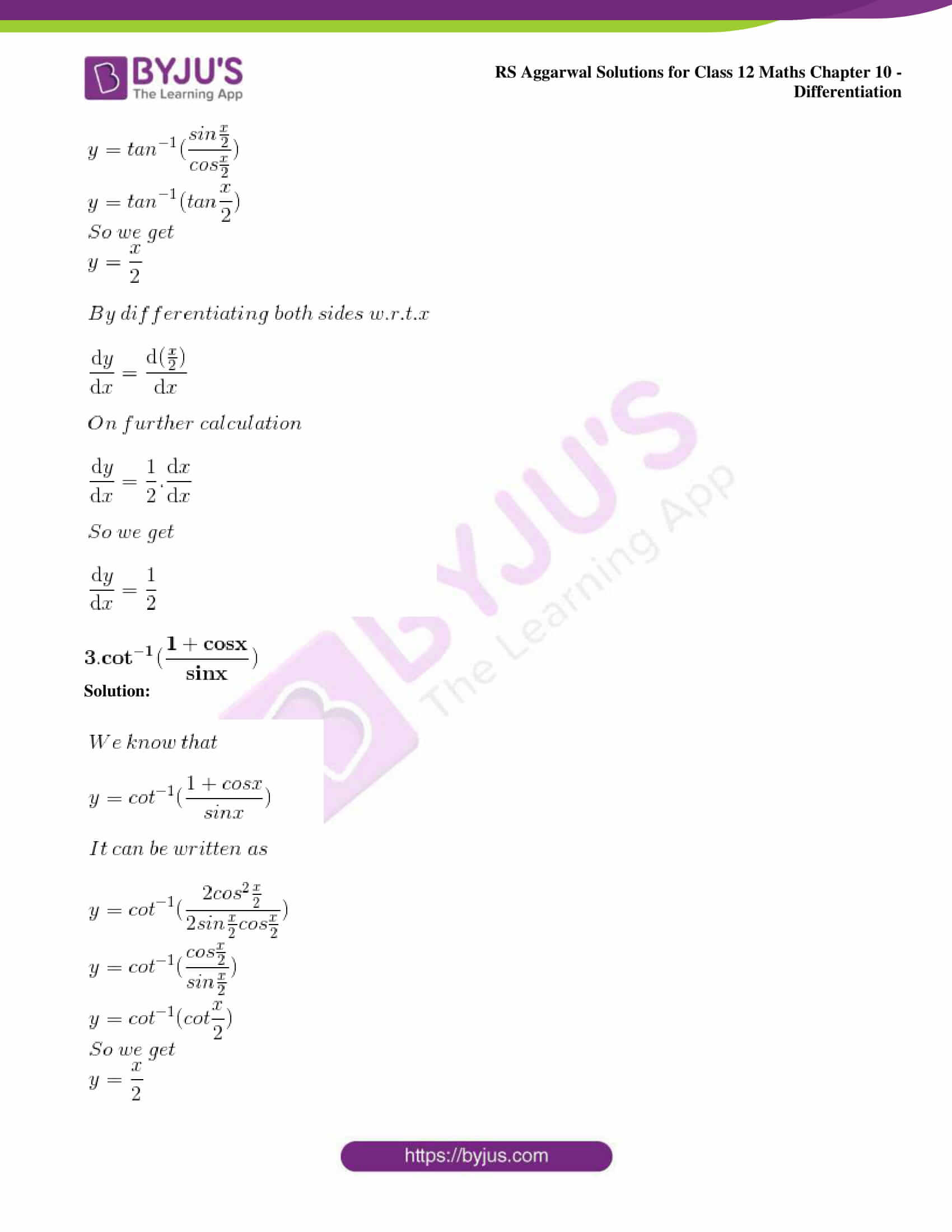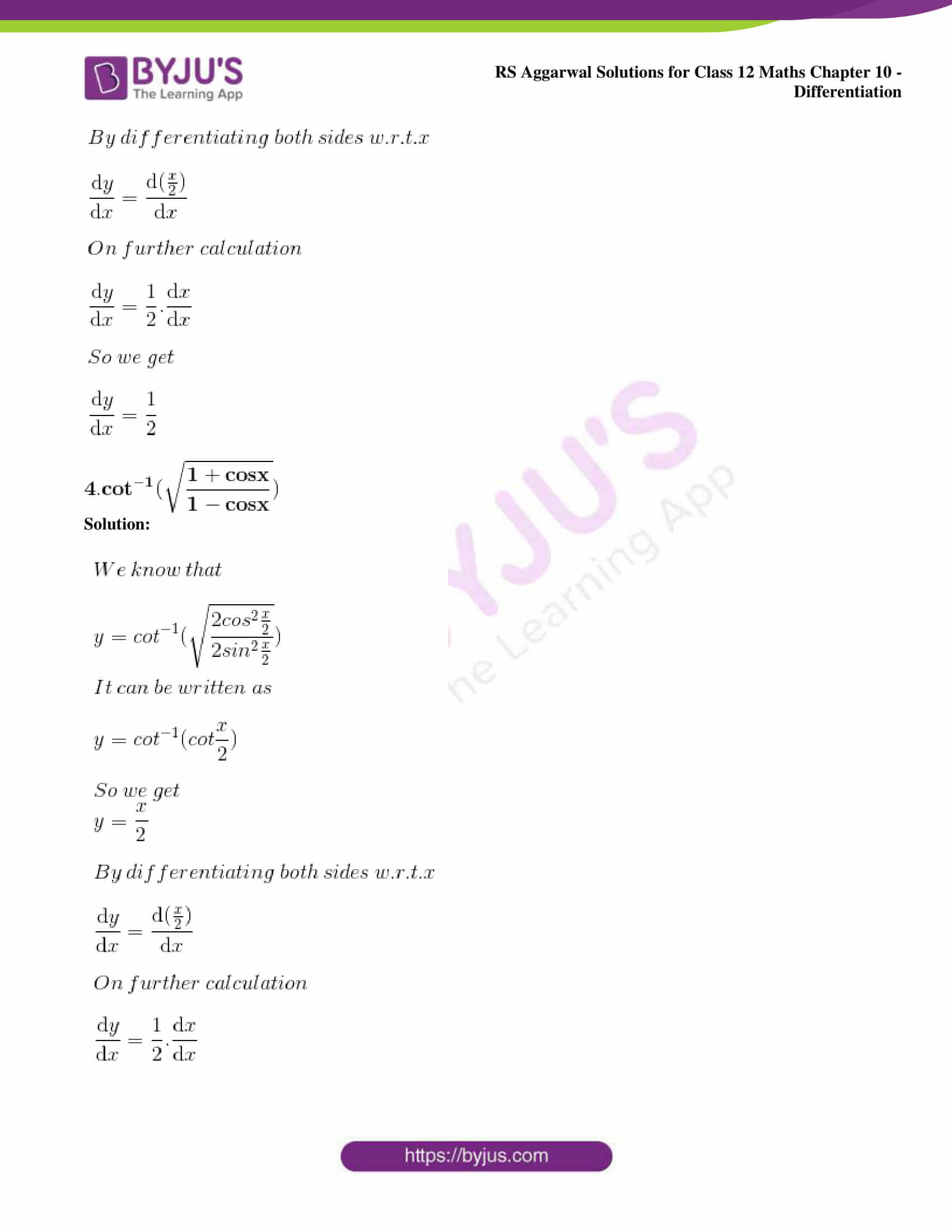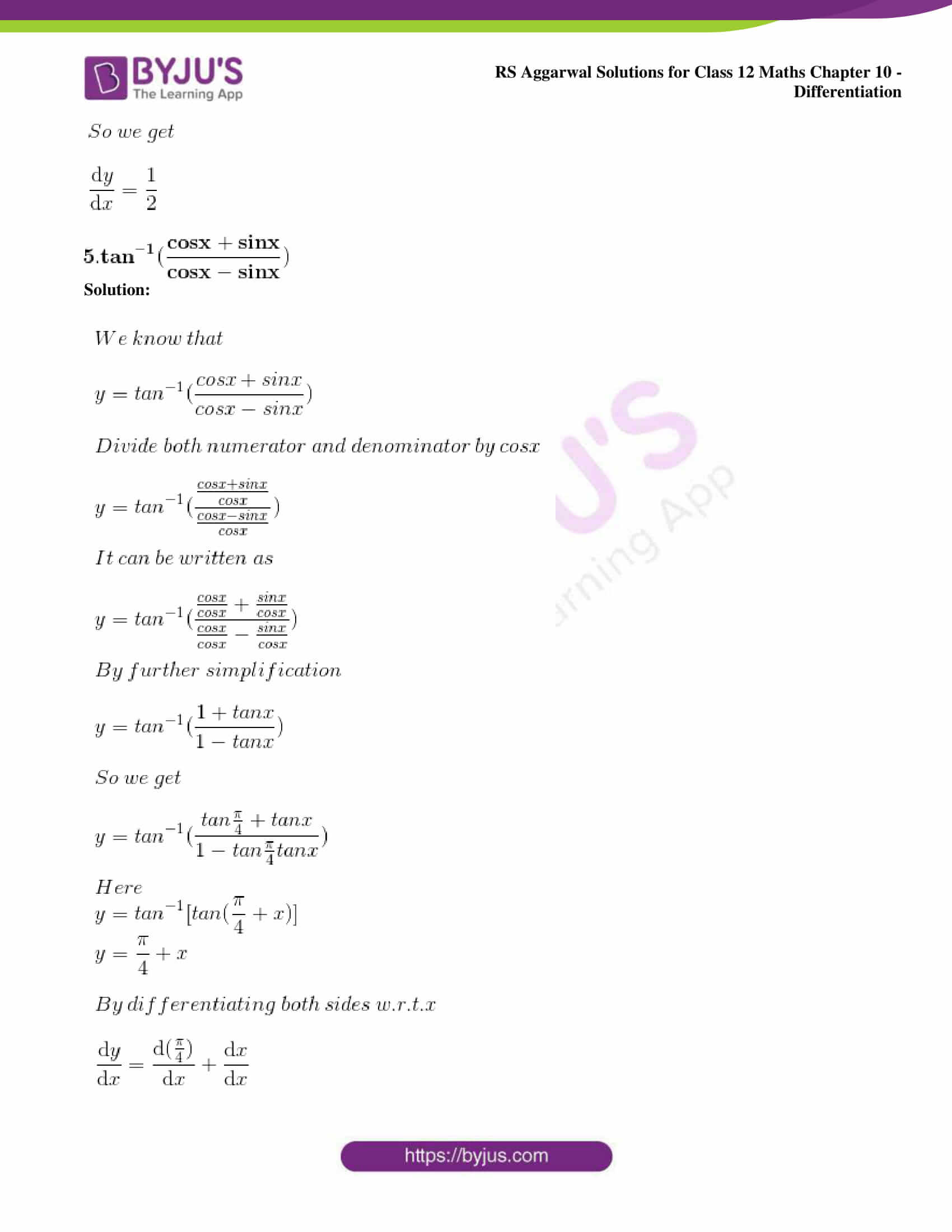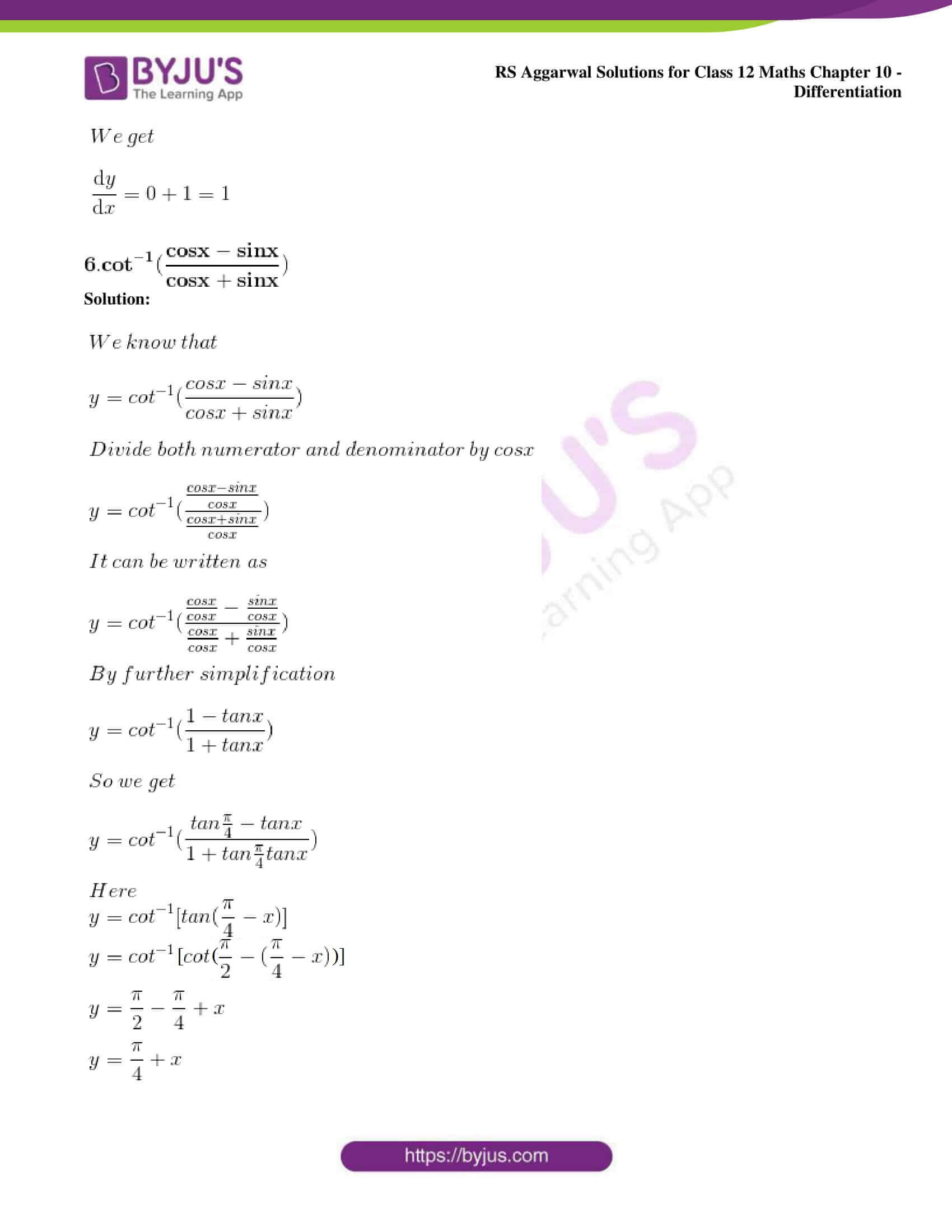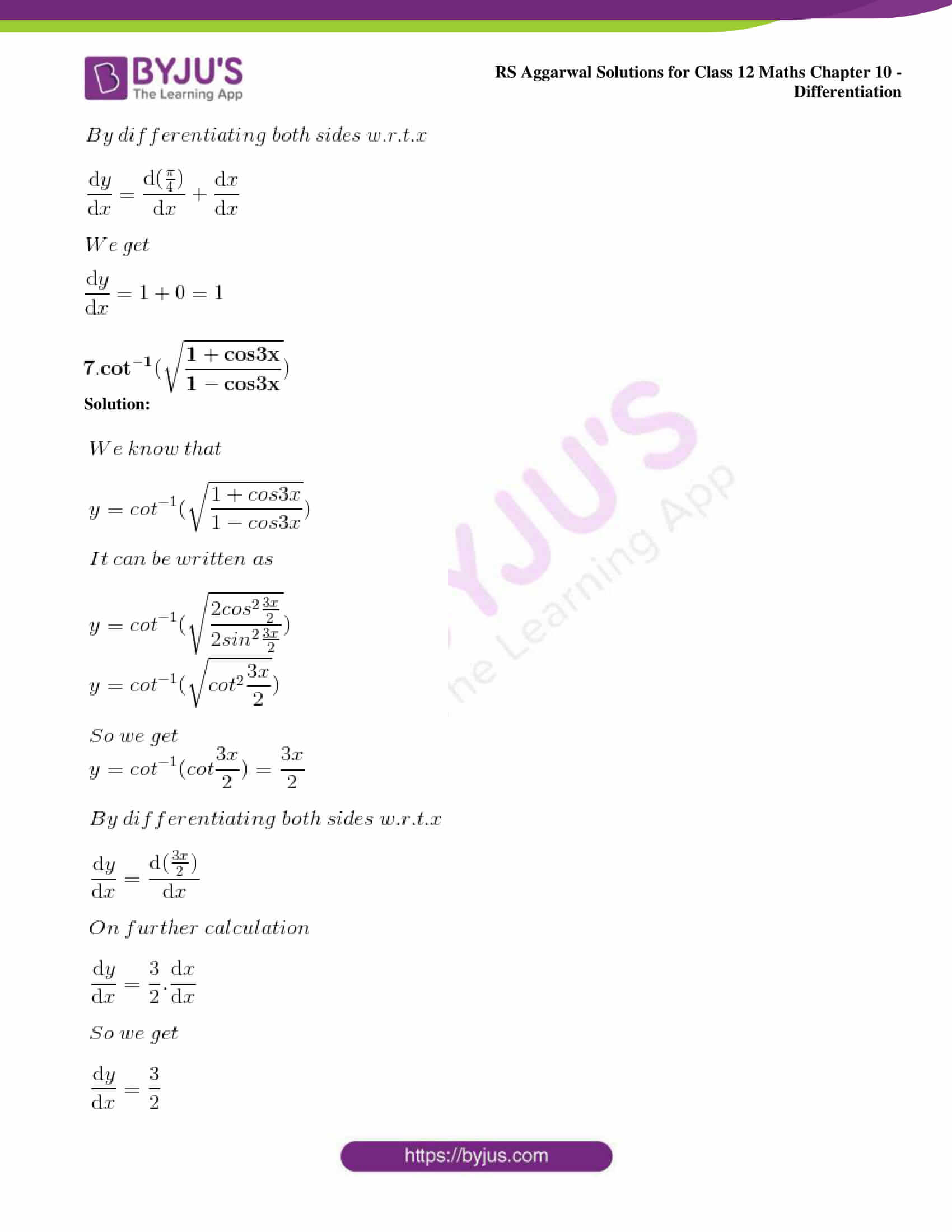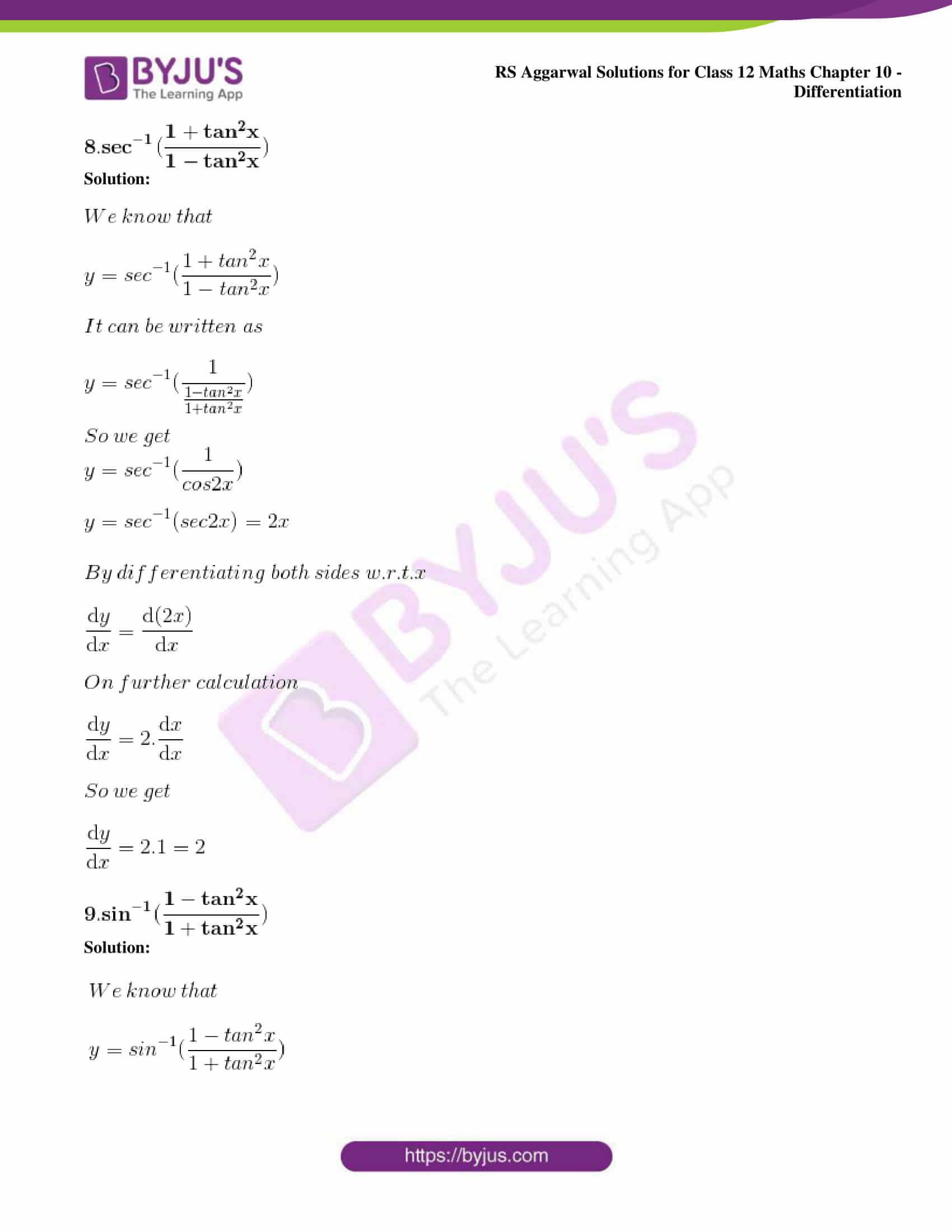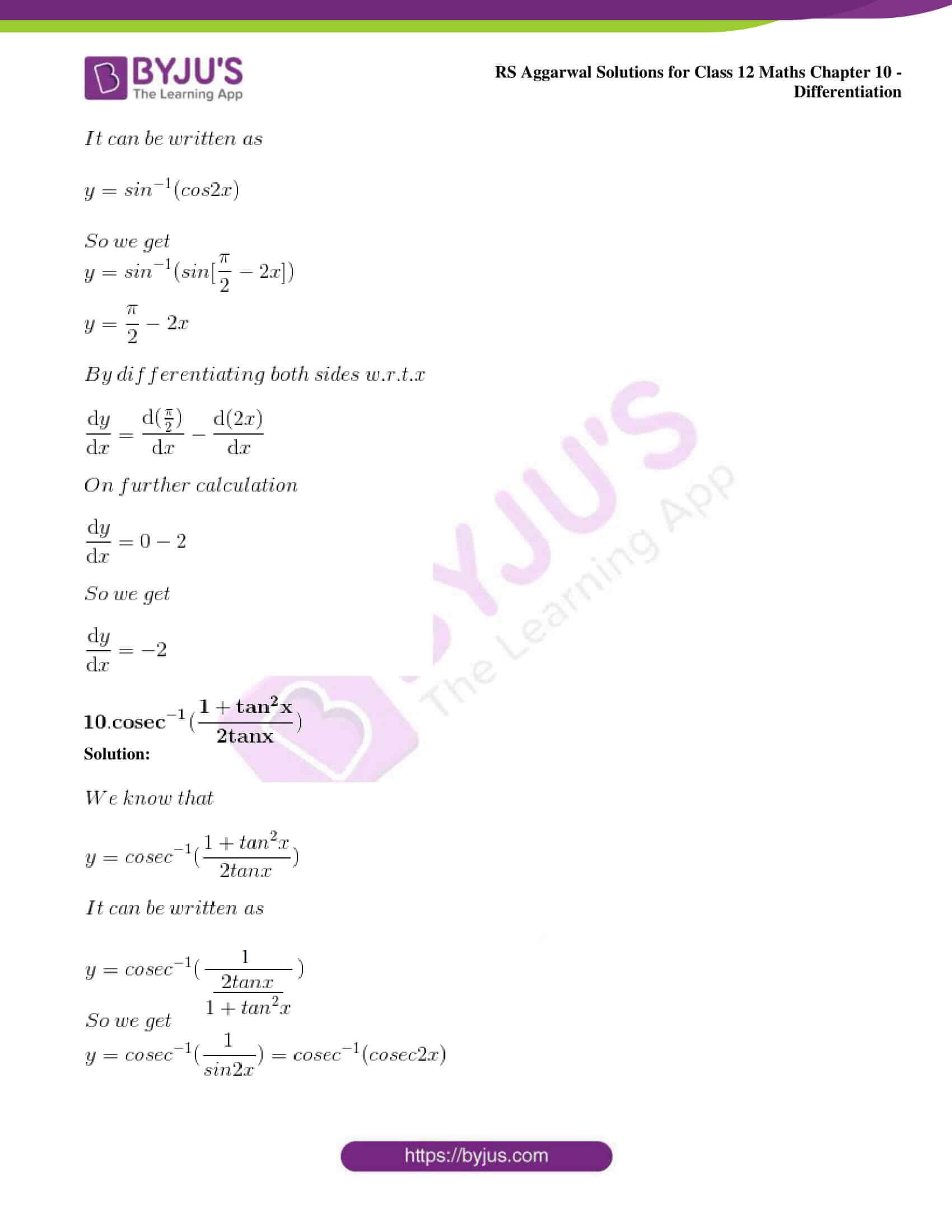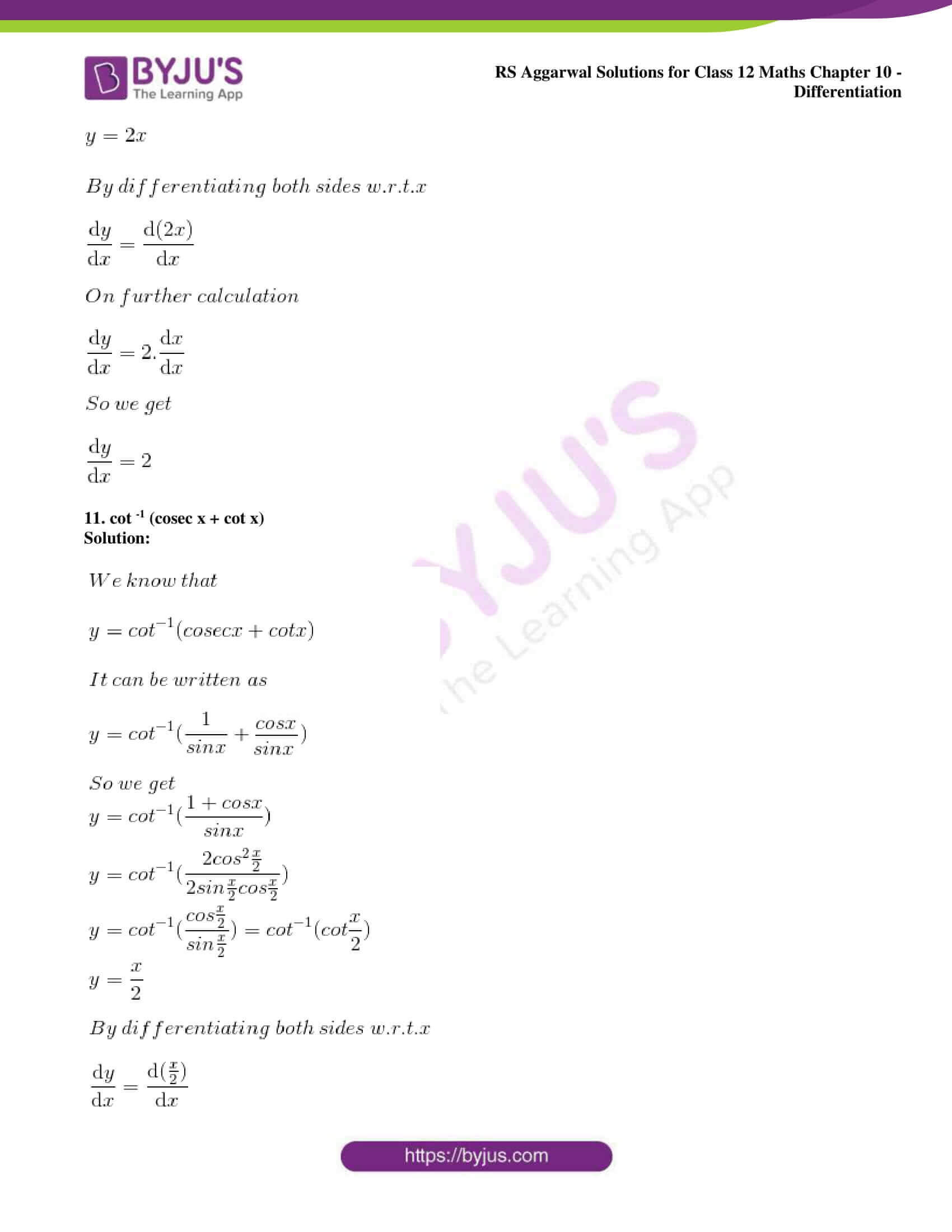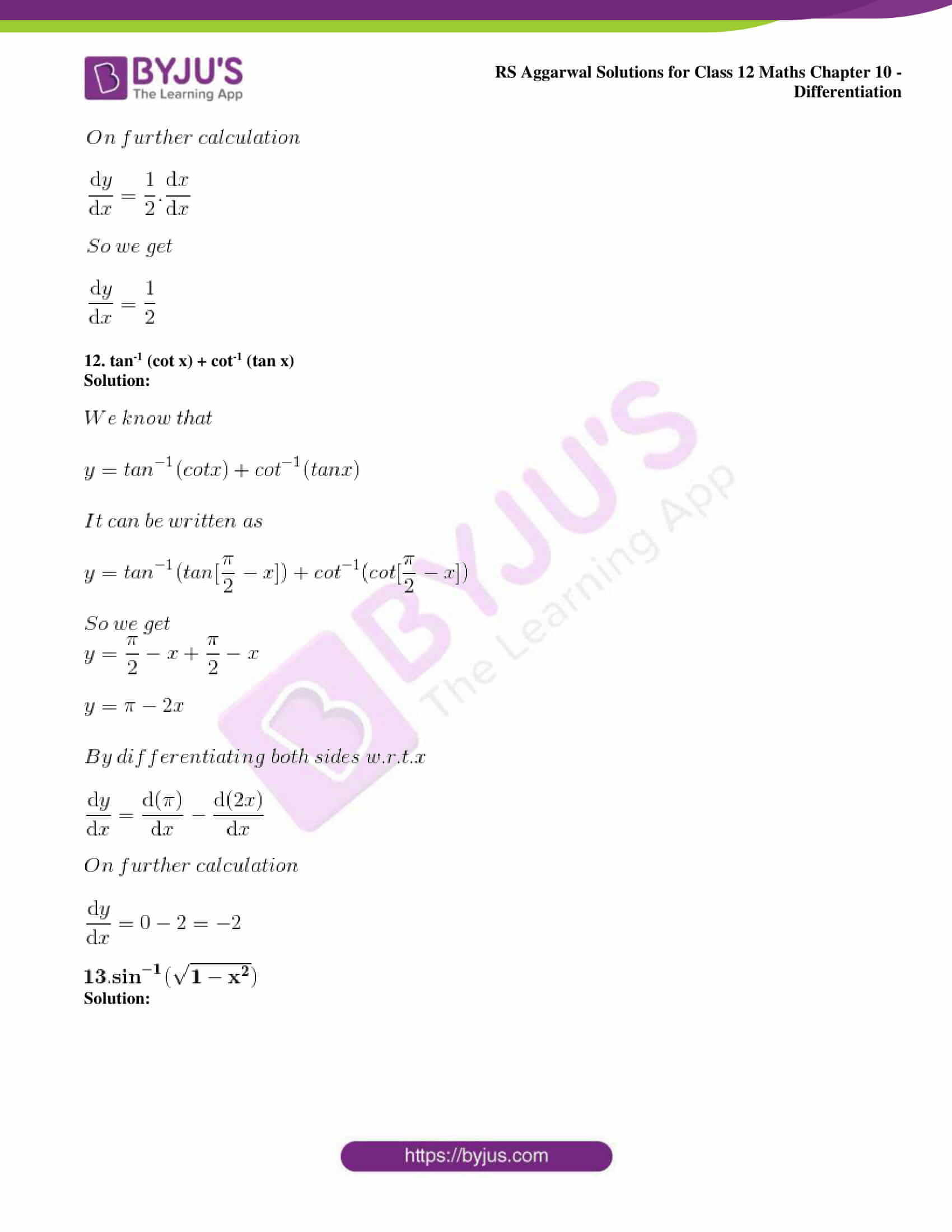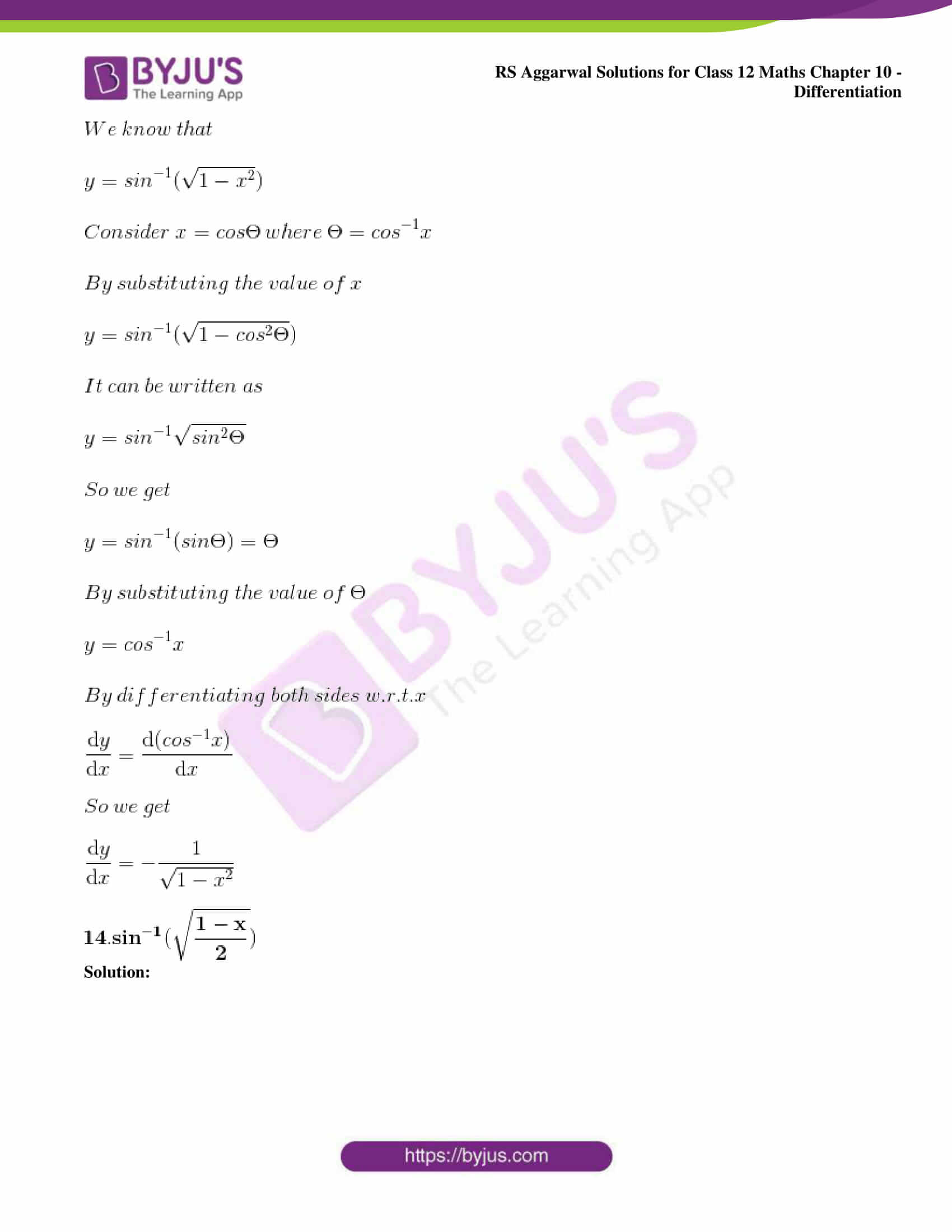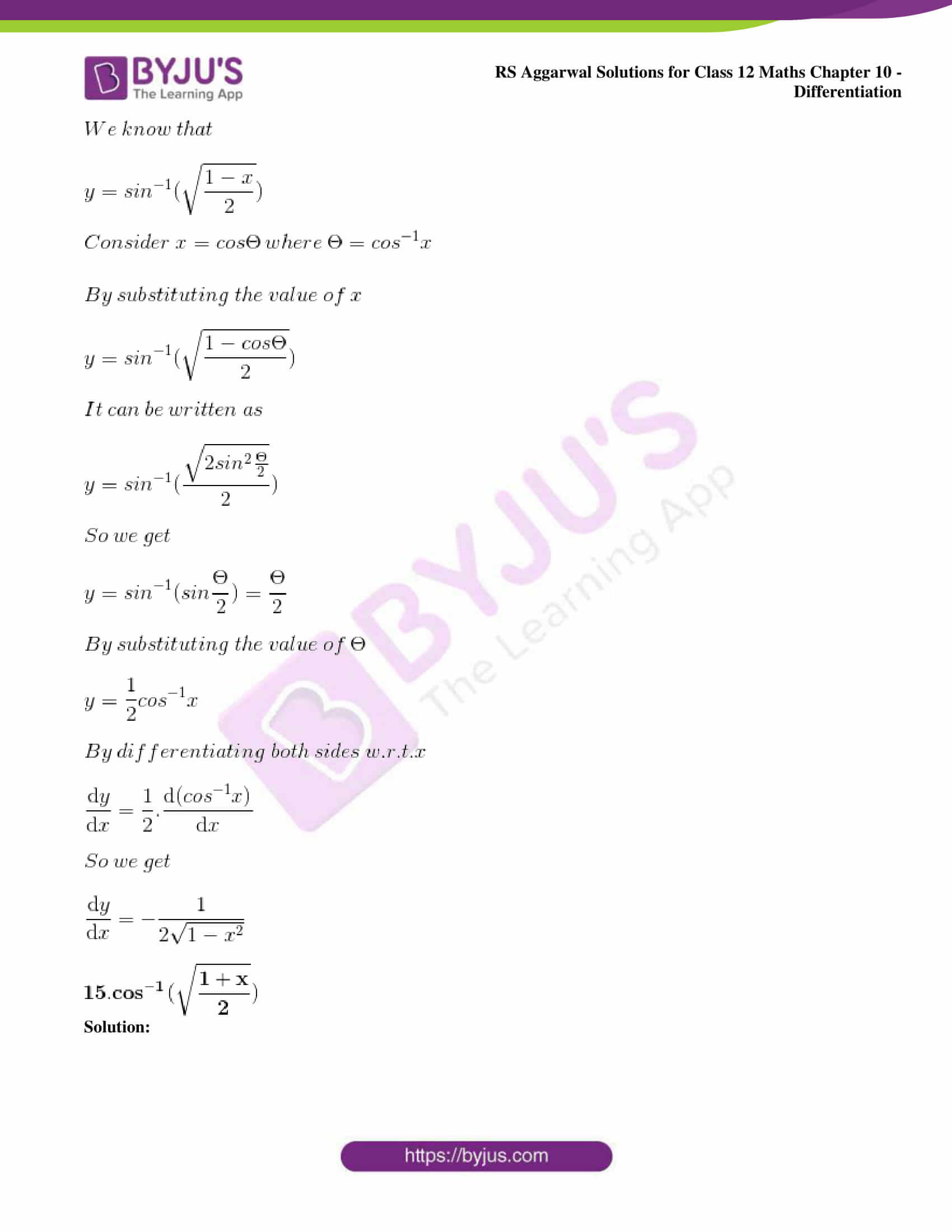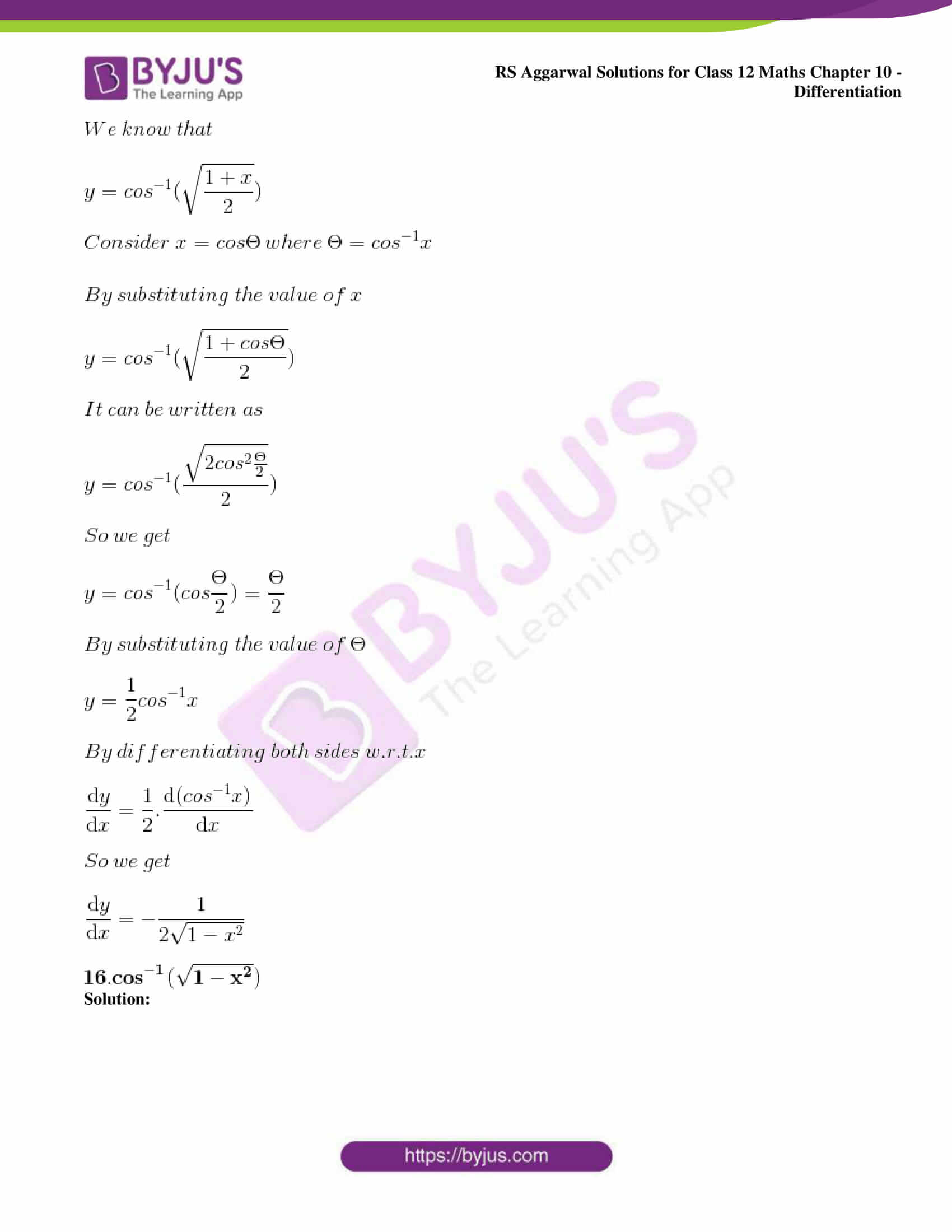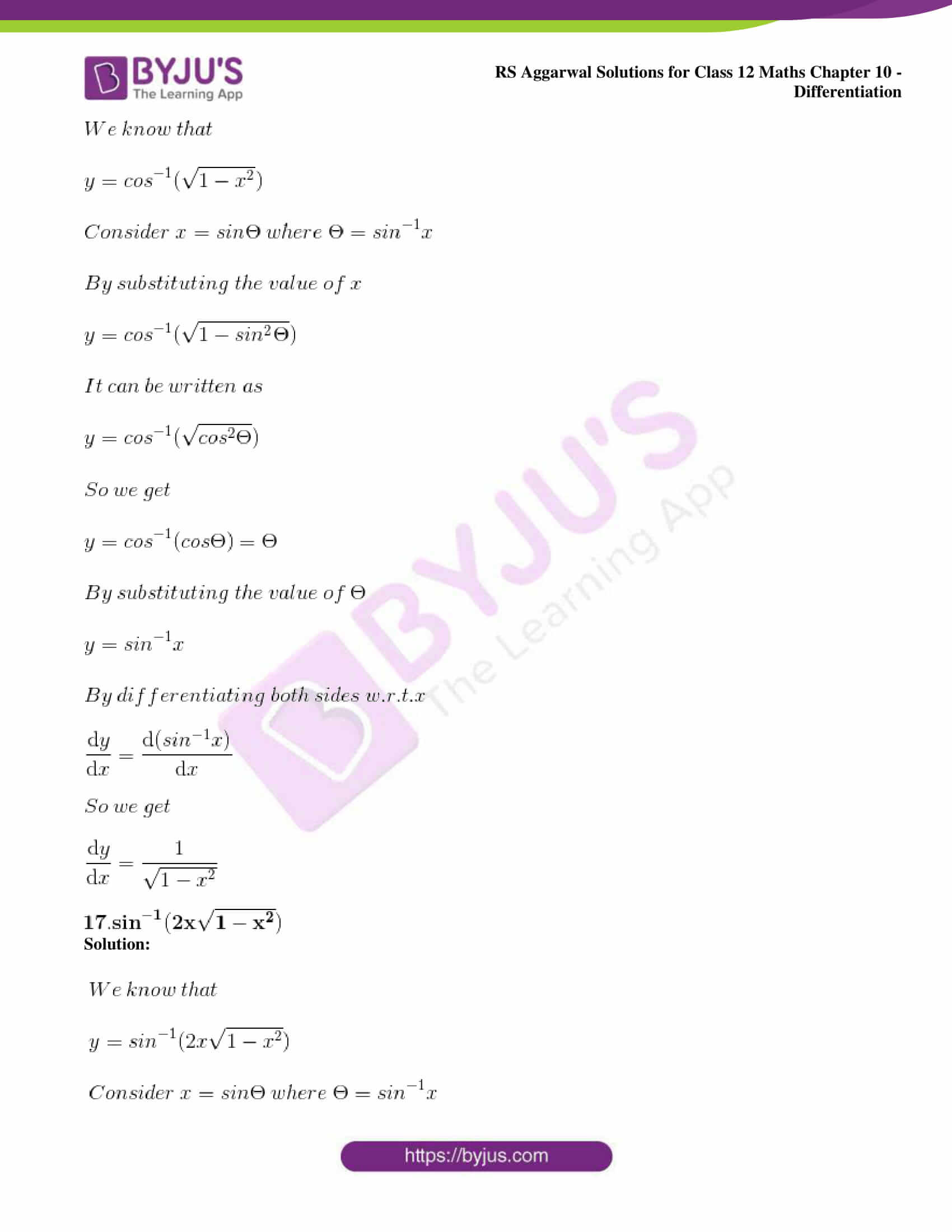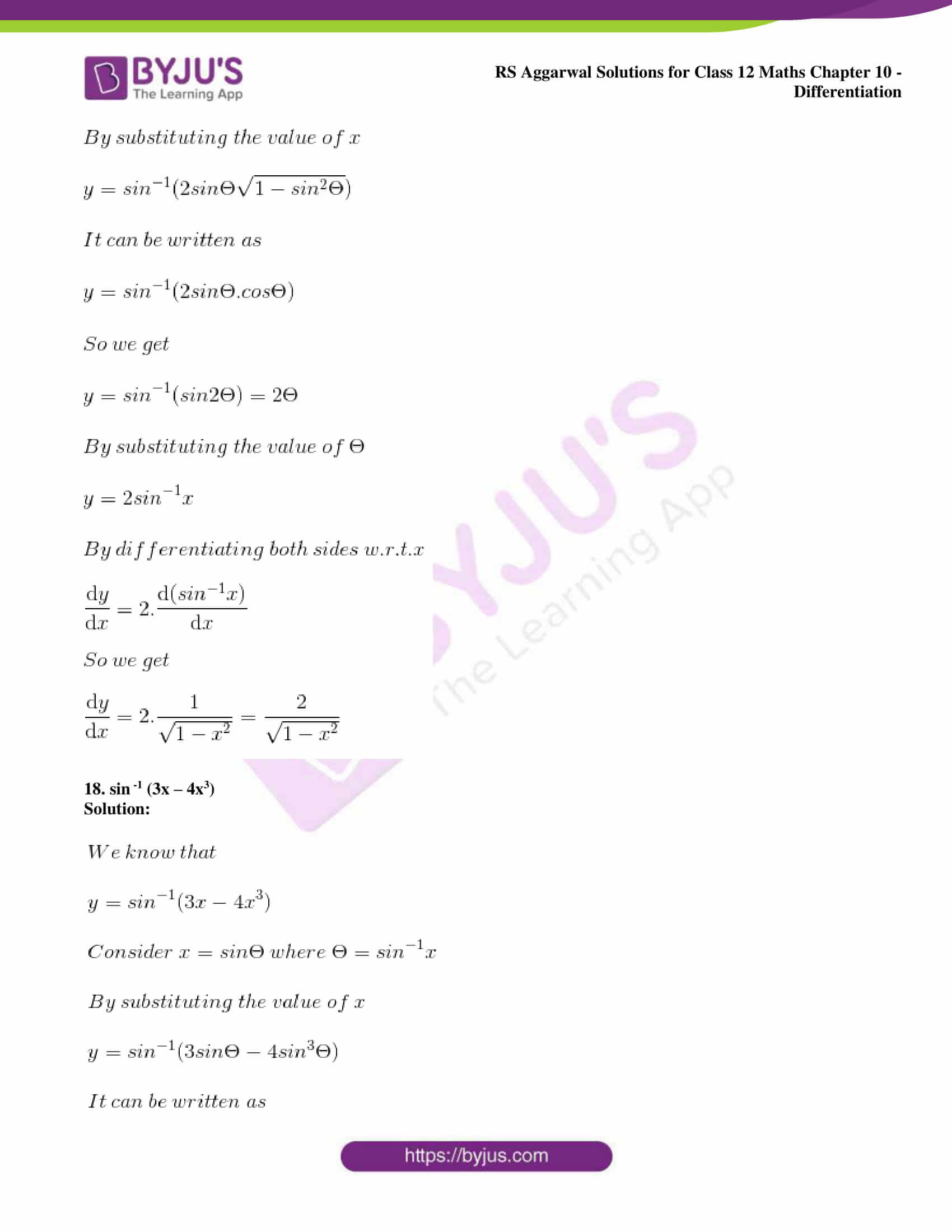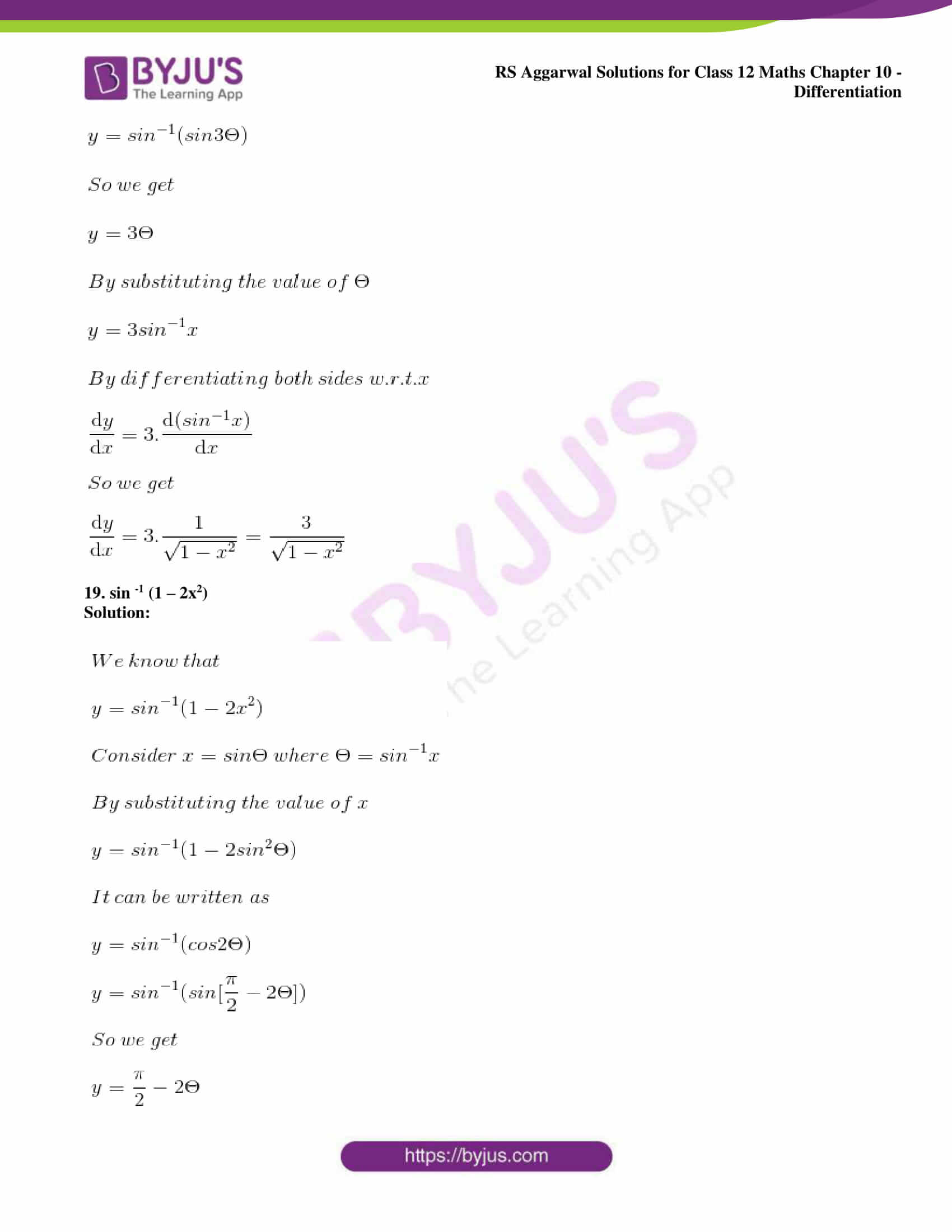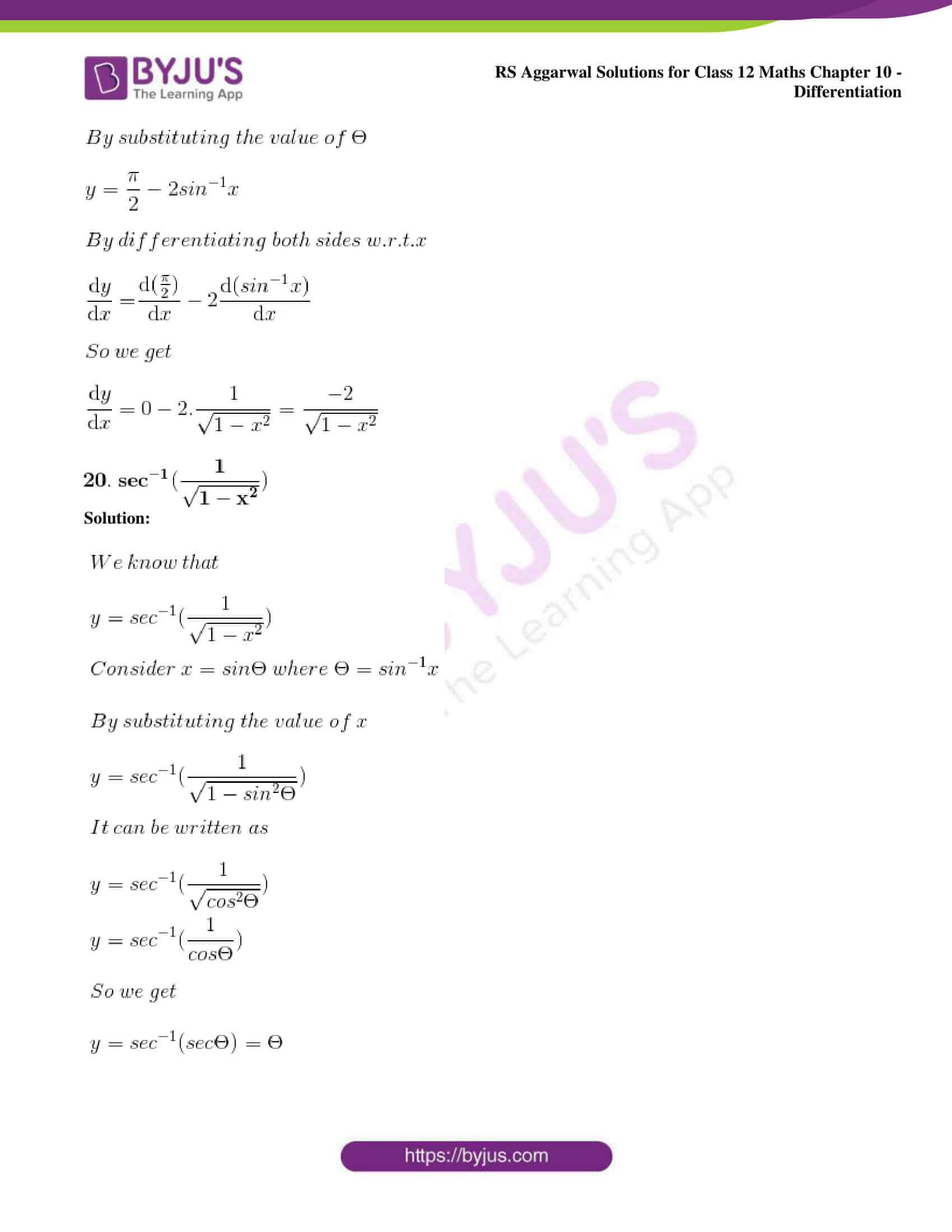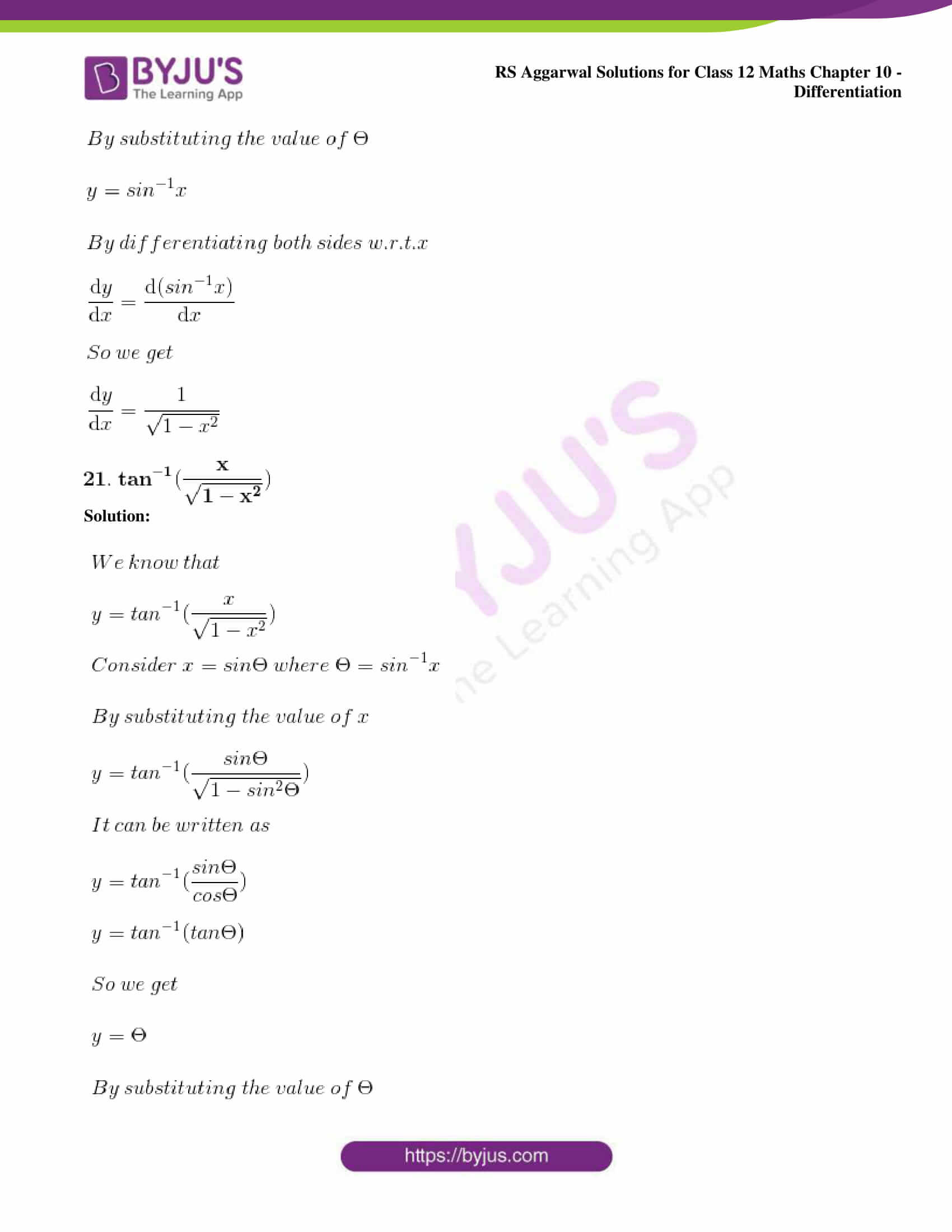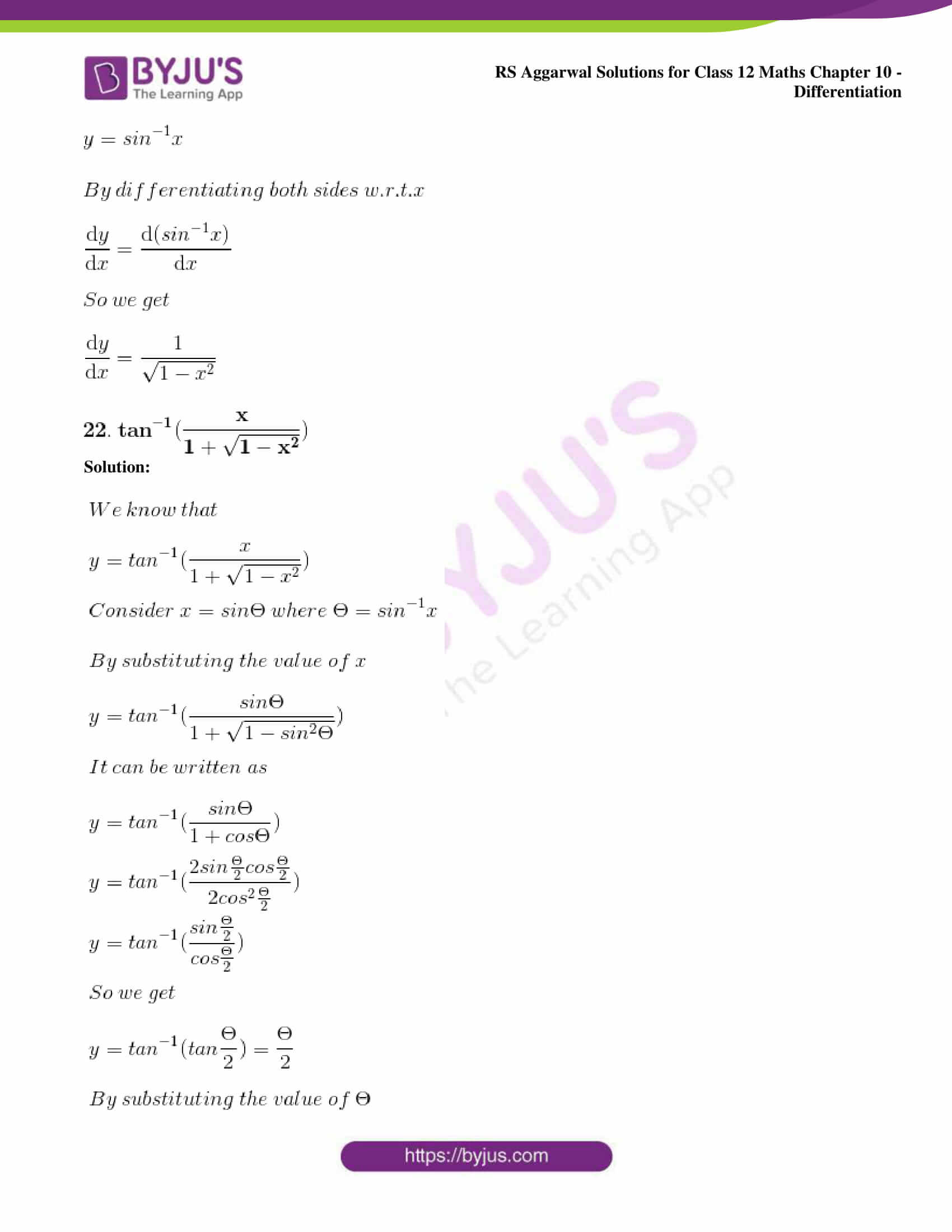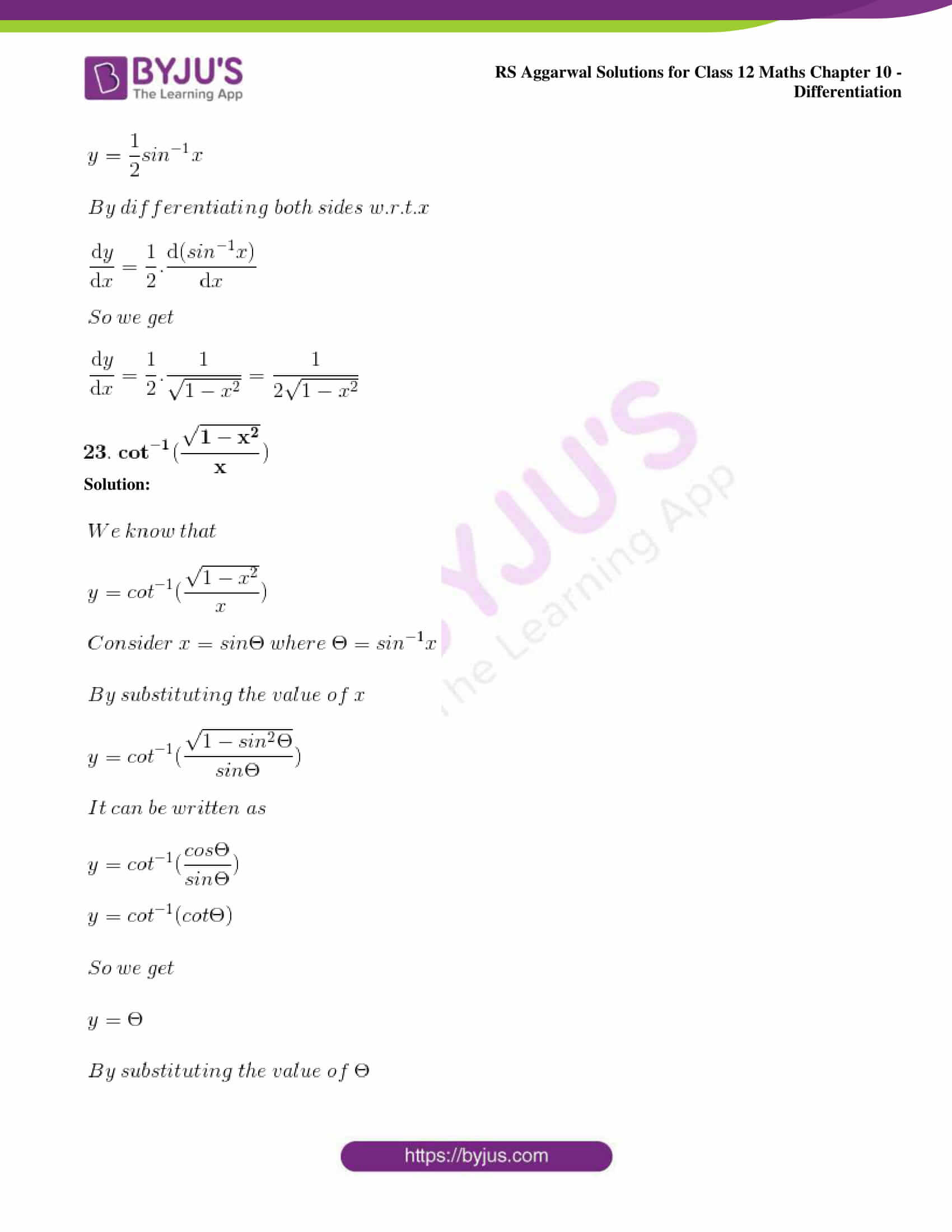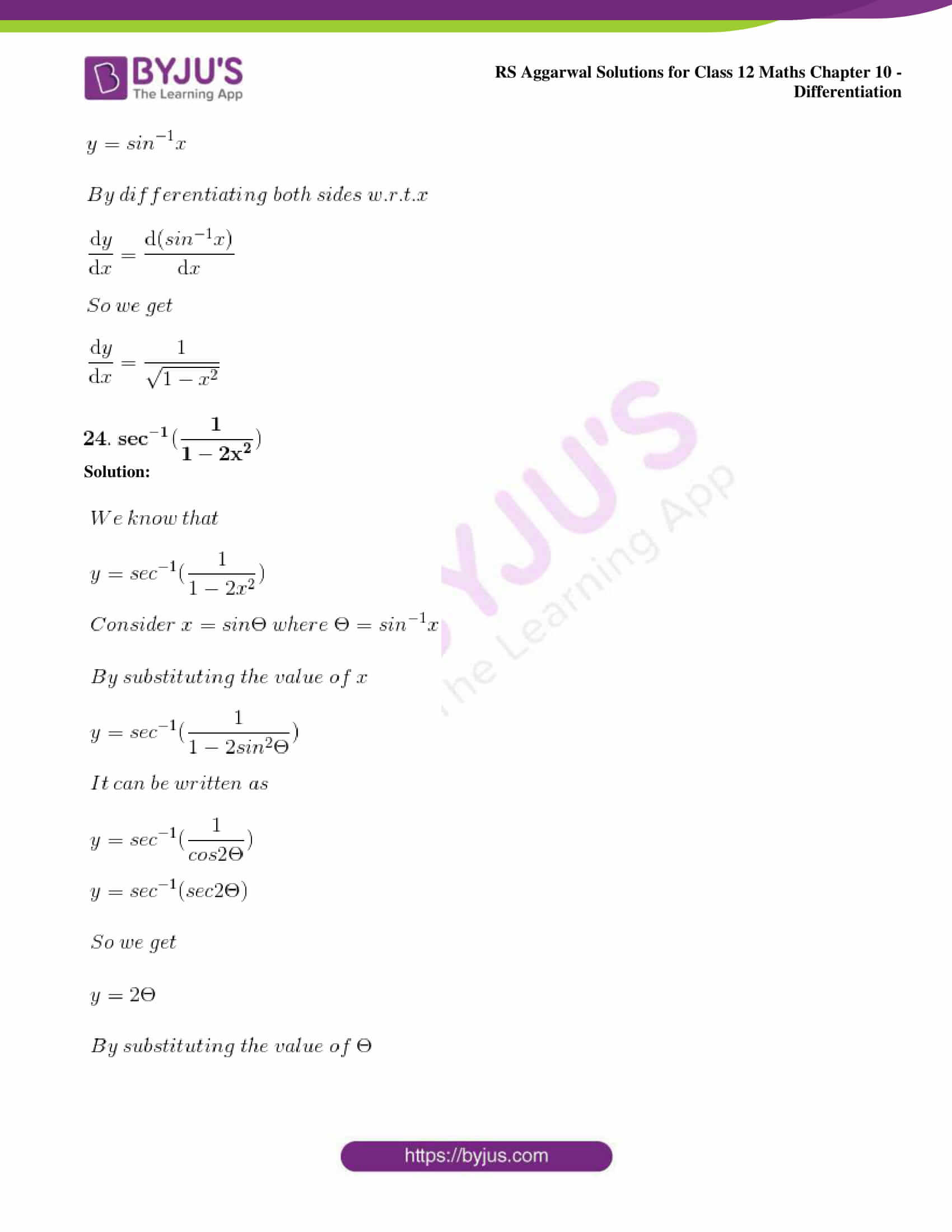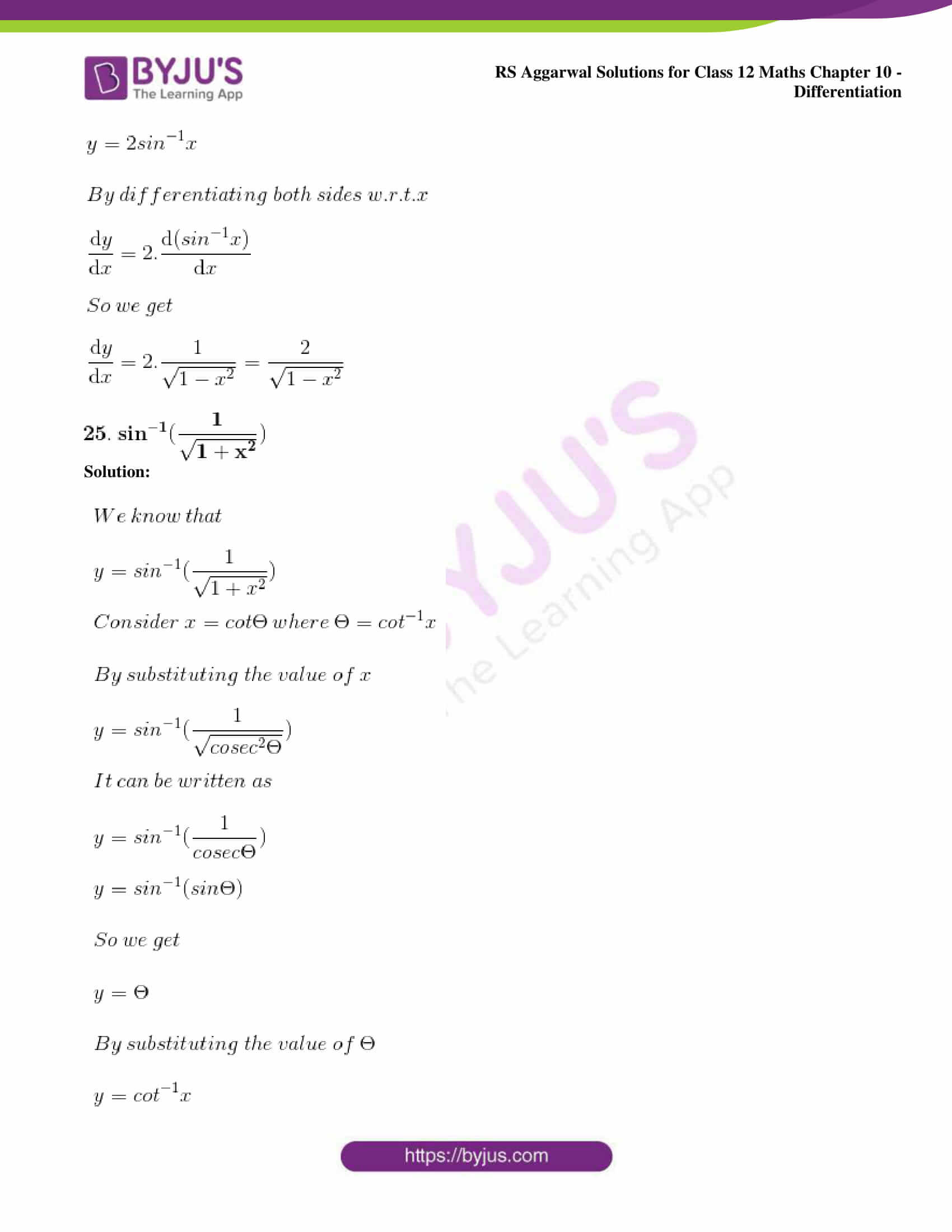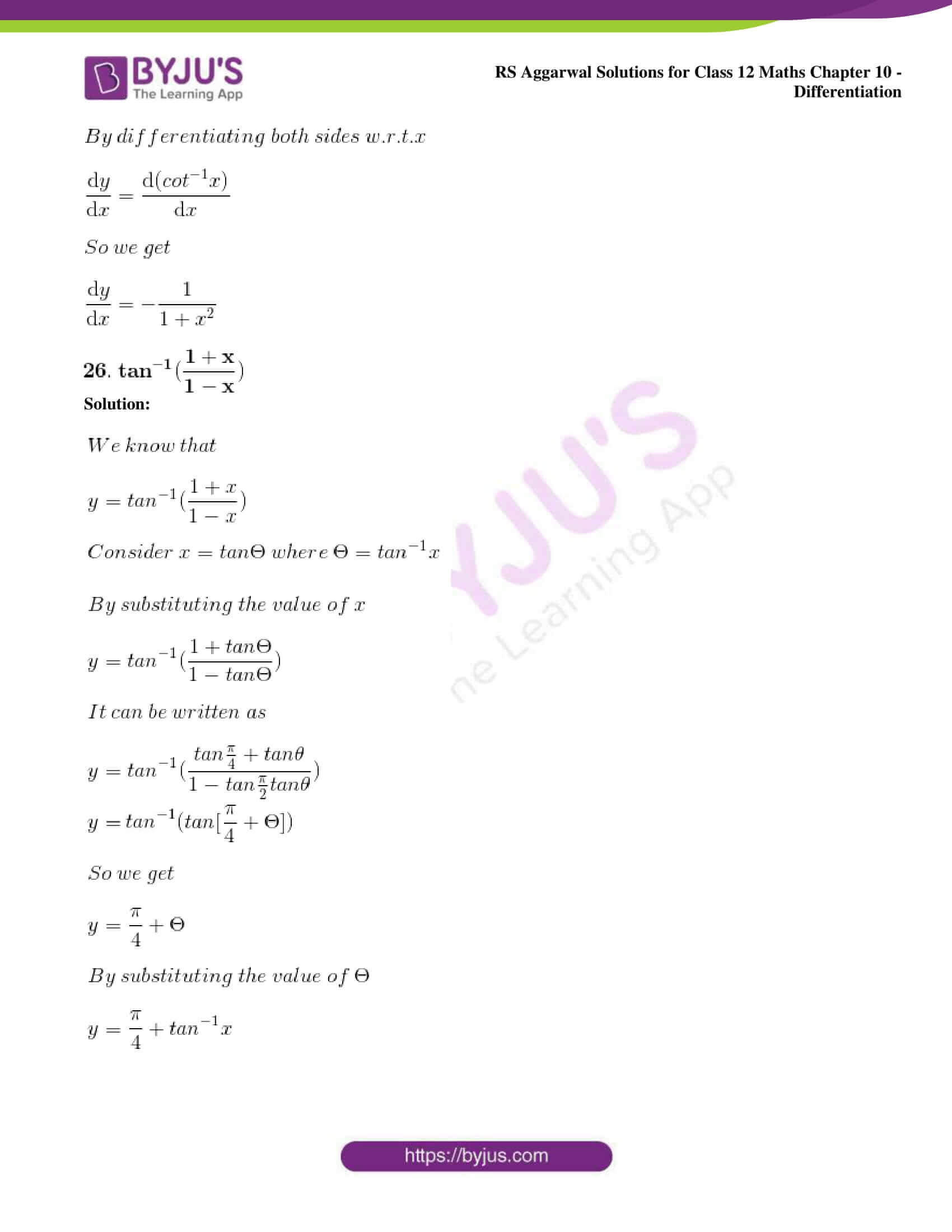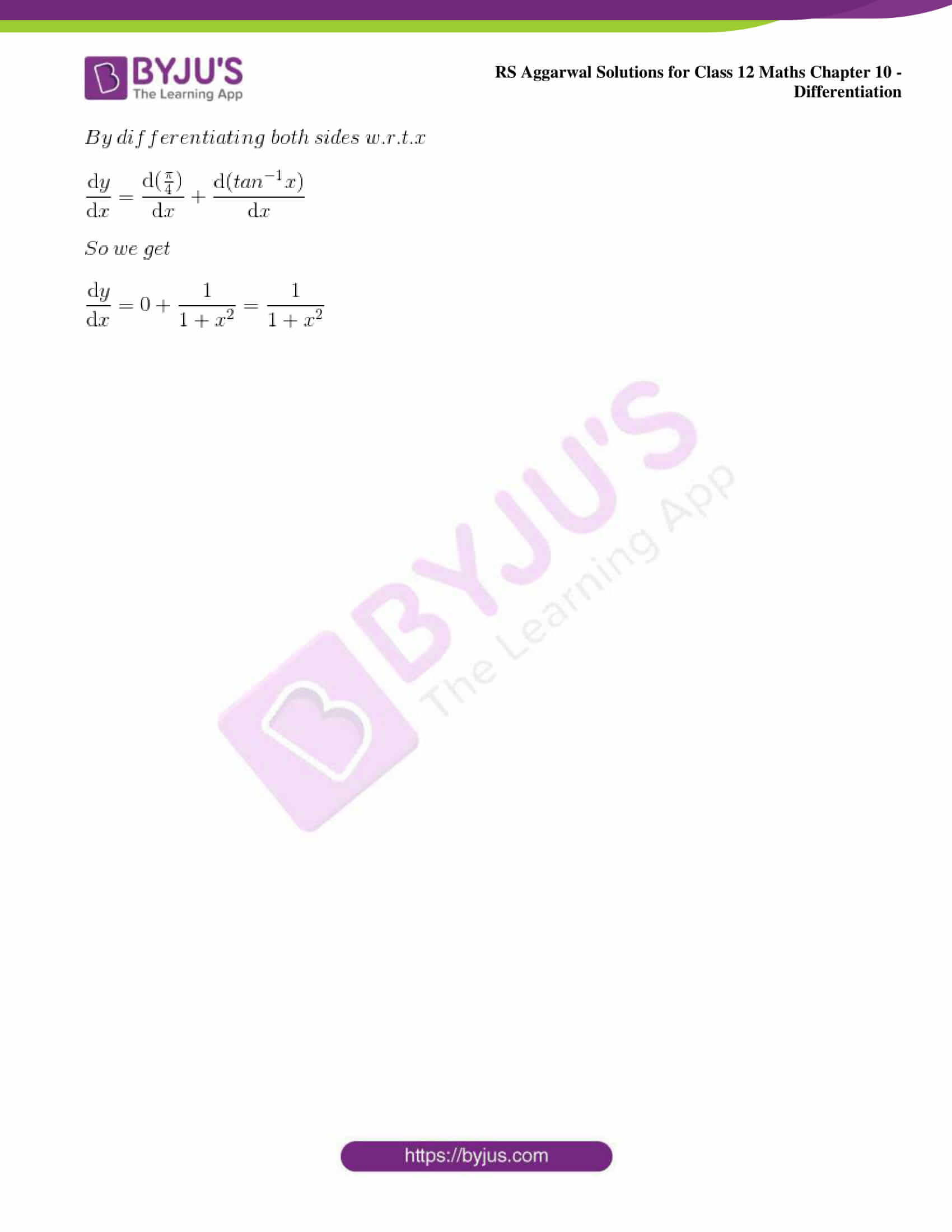### Access other exercise solutions of Class 12 Maths Chapter 10: Differentiation

Exercise 10A Solutions

Exercise 10B Solutions

Exercise 10C Solutions

Exercise 10E Solutions

Exercise 10F Solutions

Exercise 10G Solutions

Exercise 10H Solutions

Exercise 10I Solutions

Exercise 10J Solutions

### Access RS Aggarwal Solutions for Class 12 Chapter 10: Differentiation Exercise 10D

Differentiate each of the following w.r.t. x: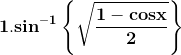Solution: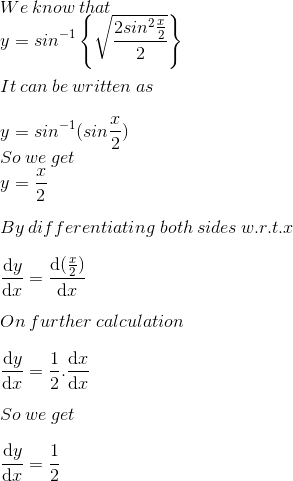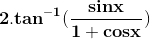Solution: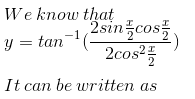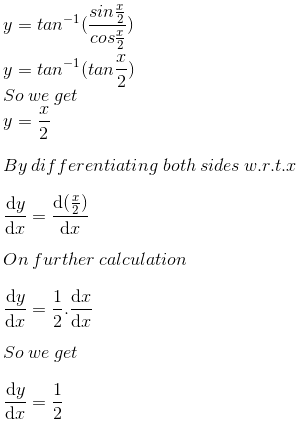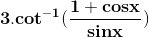Solution: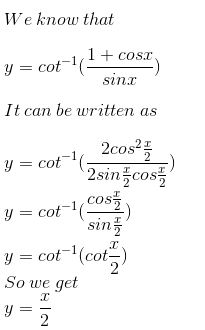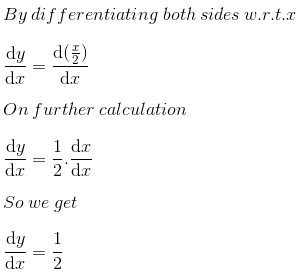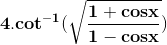Solution: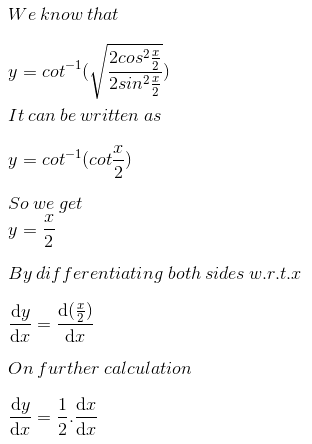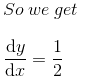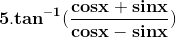Solution: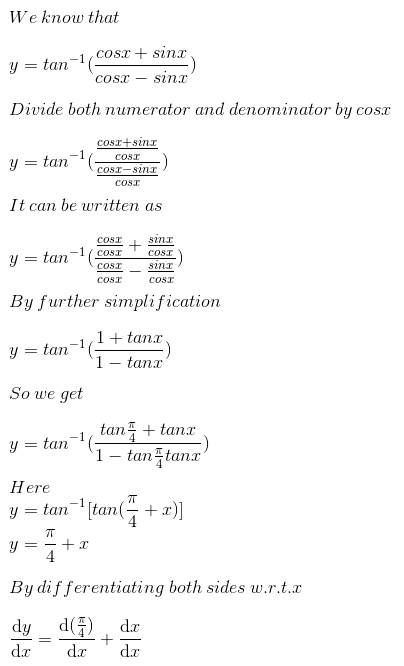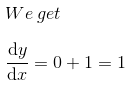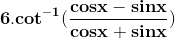Solution: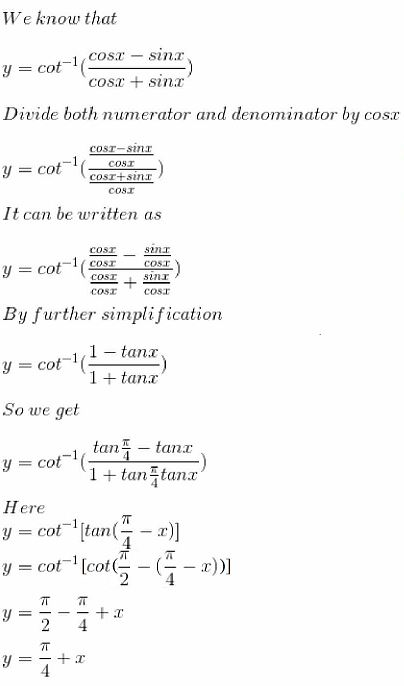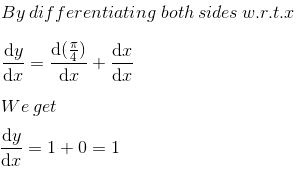Solution: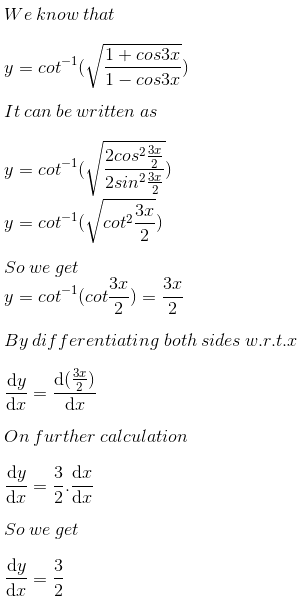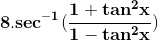Solution: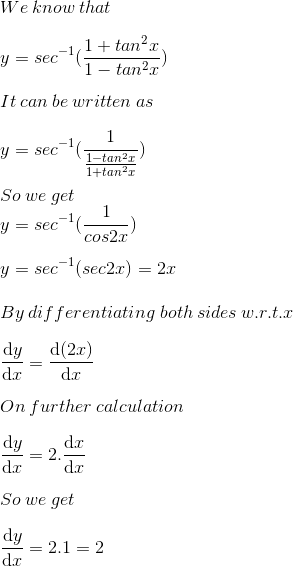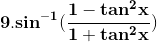Solution: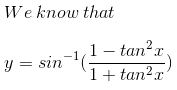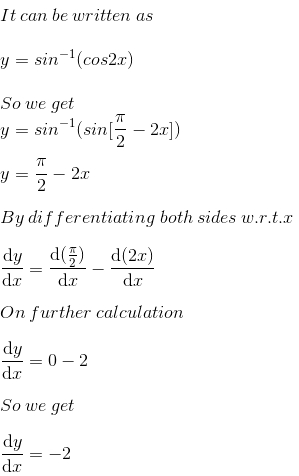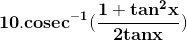Solution: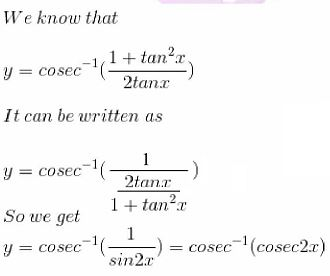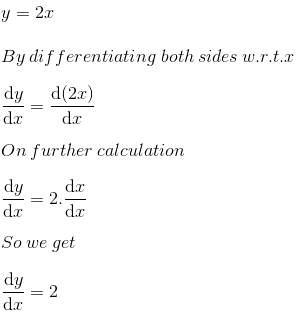11. cot -1 (cosec x + cot x)

Solution: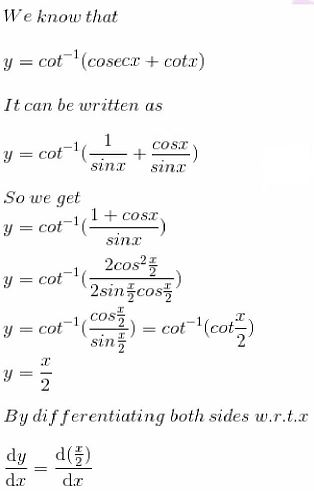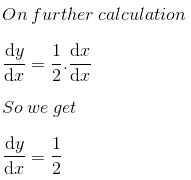12. tan-1 (cot x) + cot-1 (tan x)

Solution: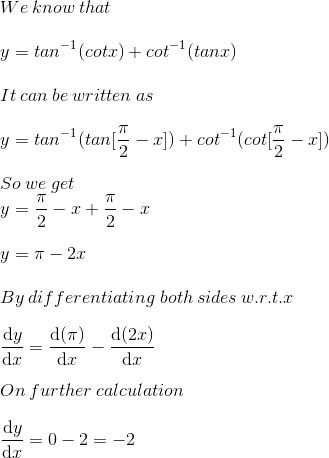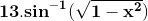Solution: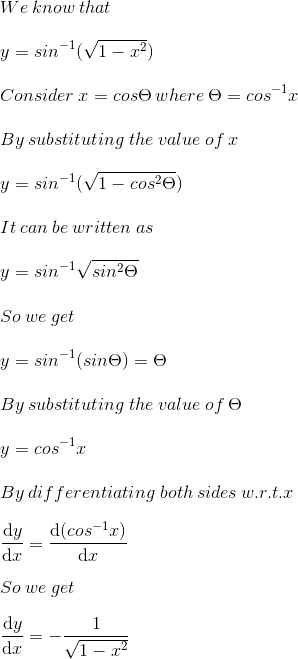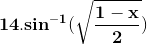Solution: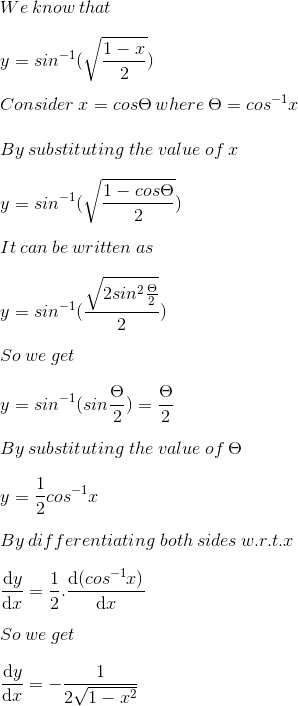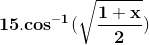Solution: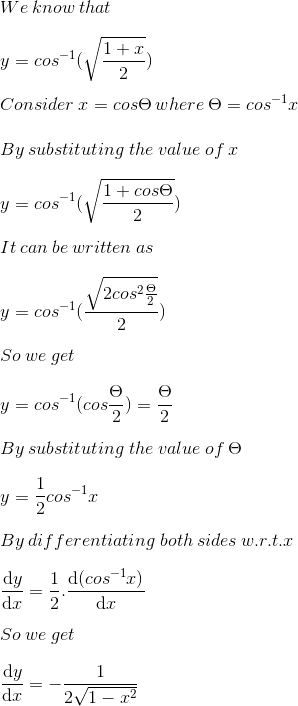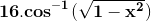Solution: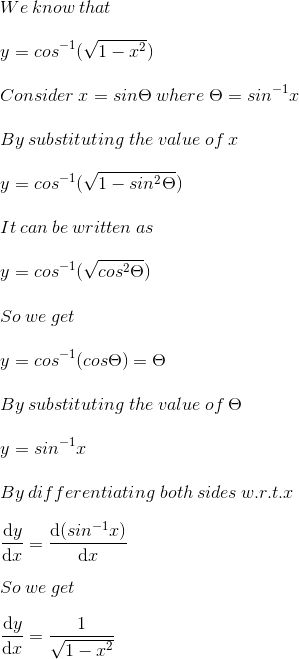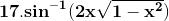Solution: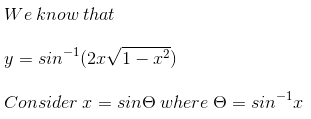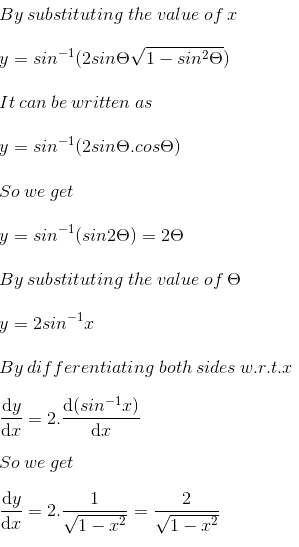18. sin -1 (3x – 4x3)

Solution: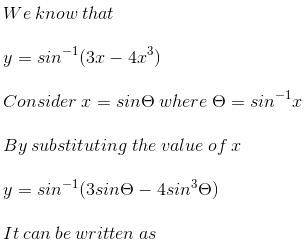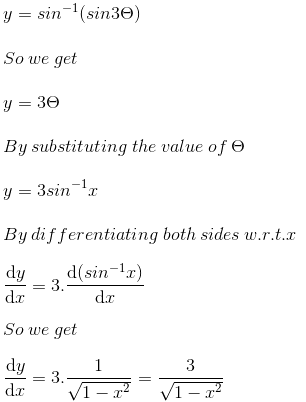19. sin -1 (1 – 2x2)

Solution: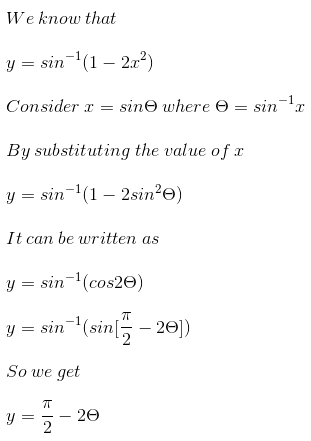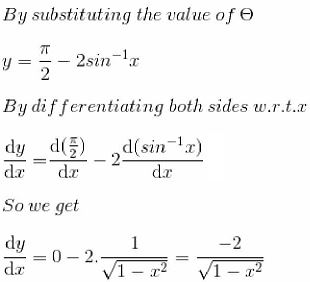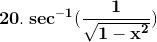Solution: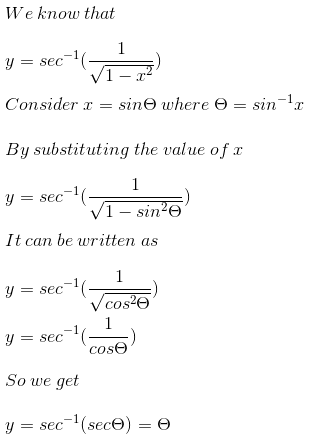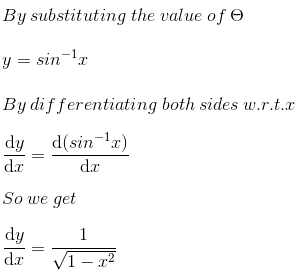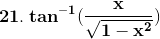Solution: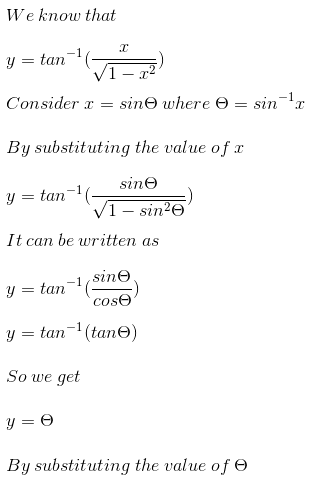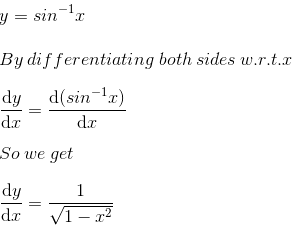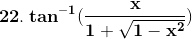Solution: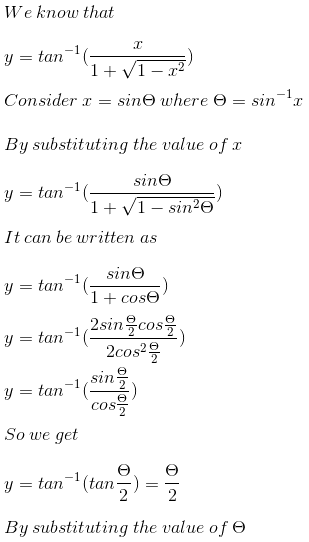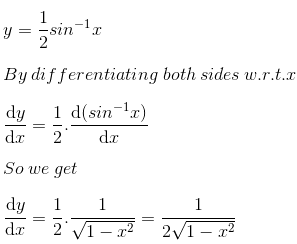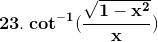Solution: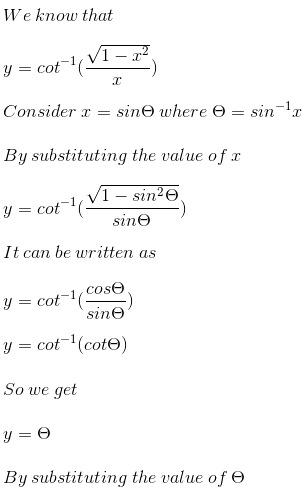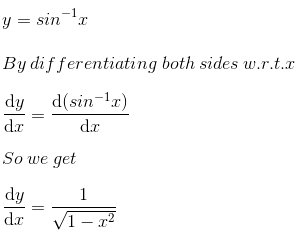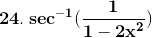Solution: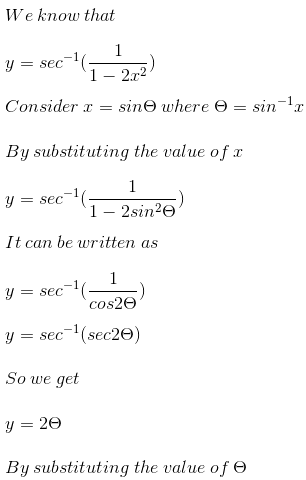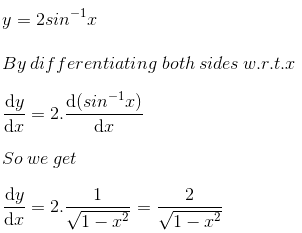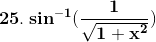Solution: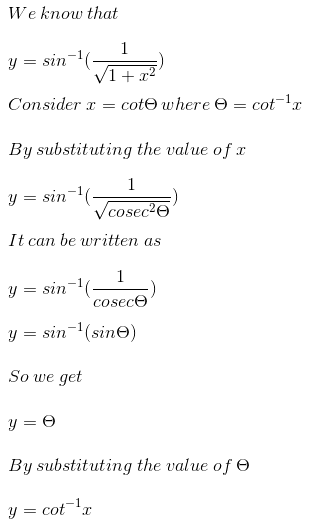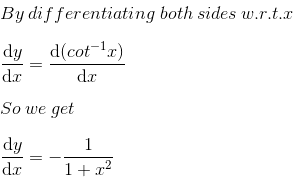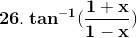Solution: